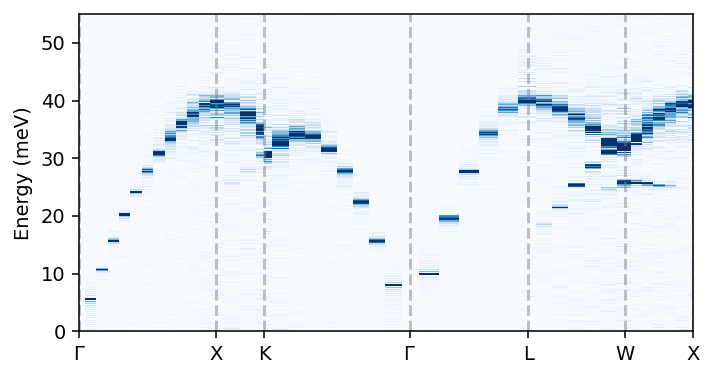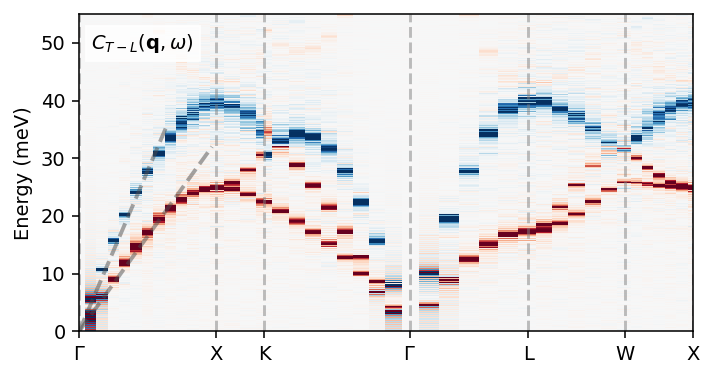# Dynamic properties of FCC-Al¶

This example demonstrate the analysis of the dynamics of crystalline aluminium in the face-centered cubic (FCC) structure via the dynamic structure factor as well as current correlation functions. Here, we use a rather small simulation cell (i.e., few q-points) and a short trajectory in order to make the tutorial run fast. In practice one needs to tune these parameters as well as the parameters used when calling dynasor for optimal results.

This example uses a trajectory that can be downloaded from zenodo via

wget https://zenodo.org/records/10014454/files/dumpT300.NVT.atom.velocity.gz


or generated from scratch by running lammps using the input files provided.

:

import numpy as np
import matplotlib.pyplot as plt
from ase import Atoms
from ase.build import bulk
from dynasor import compute_dynamic_structure_factors, Trajectory
from dynasor.qpoints import get_supercell_qpoints_along_path
from seekpath import get_path


## Set up the Trajectory¶

dynasor supports multiple different trajectory formats via internal readers as well as via mdanalysis and ase. Here, we use the internal lammps reader.

:

trajectory_filename = 'dumpT300.NVT.atom.velocity.gz'
traj = Trajectory(trajectory_filename,
trajectory_format='lammps_internal', frame_stop=10000)

INFO: Trajectory file: dumpT300.NVT.atom.velocity.gz
INFO: Total number of particles: 6912
INFO: Number of atom types: 1
INFO: Number of atoms of type X: 6912
INFO: Simulation cell (in Angstrom):
[[48.78  0.    0.  ]
[ 0.   48.78  0.  ]
[ 0.    0.   48.78]]


A short summary of the content of the traj object can be obtained via the display (in notebooks) or print (in notebooks or scripts) command.

:

display(traj)


### Trajectory

FieldValue
File namedumpT300.NVT.atom.velocity.gz
Number of atoms6912
Cell metric[[48.78 0. 0. ] [ 0. 48.78 0. ] [ 0. 0. 48.78]]
Frame step1
Atom types['X']

## Set up path through Brillouin zone¶

We now need to define the path through the Brillouin zone that we want to sample. The latter can be generated automatically for the crystal structure of interest using the seekpath package. To find the q-points along this path that can be sampled using the supercell used in the molecular dynamics simulations we use the function get_supercell_qpoints_along_path.

The primitive structure is generate using the bulk function from the ase package.

:

prim = bulk('Al', a=4.065)
path_info = get_path((
prim.cell,
prim.get_scaled_positions(),
prim.numbers))
point_coordinates = path_info['point_coords']
path = path_info['path']
display(point_coordinates)
display(path)

{'GAMMA': [0.0, 0.0, 0.0],
'X': [0.5, 0.0, 0.5],
'L': [0.5, 0.5, 0.5],
'W': [0.5, 0.25, 0.75],
'W_2': [0.75, 0.25, 0.5],
'K': [0.375, 0.375, 0.75],
'U': [0.625, 0.25, 0.625]}

[('GAMMA', 'X'),
('X', 'U'),
('K', 'GAMMA'),
('GAMMA', 'L'),
('L', 'W'),
('W', 'X')]

:

q_segments = get_supercell_qpoints_along_path(
path, point_coordinates, prim.cell, traj.cell)
q_points = np.vstack(q_segments)


## Run calculation of correlation functions¶

We are now all set for calculating the dynamic structure factor as well as the current correlation functions, where the latter is triggered by setting include_currents=True. To obtain the correct units, the spacing between the frames (dt) must be specified in units of femtoseconds.

The following cell should take ten to twenty minutes to run on a common work station.

Note: The Trajectory object is an “exhaustible iterable” , which means that once we have iterated over all frames, it is exhausted. Therefore, one needs to “reload” the trajectory before calling compute_dynamic_structure_factors again to ensure that the entire trajectory is available for analysis.

:

%%time
sample = compute_dynamic_structure_factors(
traj, q_points, dt=25.0, window_size=500,
window_step=50, calculate_currents=True)

INFO: Spacing between samples (frame_step): 1
INFO: Time between consecutive frames in input trajectory (dt): 25.0 fs
INFO: Time between consecutive frames used (dt * frame_step): 25.0 fs
INFO: Time window size (dt * frame_step * window_size): 12500.0 fs
INFO: Angular frequency resolution: dw = 0.000503 2pi/fs = 0.331 meV
INFO: Maximum angular frequency (dw * window_size): 0.251327 2pi/fs = 165.427 meV
INFO: Nyquist angular frequency (2pi / frame_step / dt / 2): 0.126 2pi/fs = 82.7 meV
INFO: Calculating current (velocity) correlations
INFO: Number of q-points: 48
CPU times: user 17min 52s, sys: 1.04 s, total: 17min 53s
Wall time: 4min 45s


As in the case of the trajectory, we can use the display or print commands to obtain a quick summary of the Sample object returned by the compute_dynamic_structure_factors function.

:

display(sample)


### DynamicSample

FieldContent/Size
Atom types['X']
Pairs[('X', 'X')]
Particle counts{'X': 6912}
Simulations cell[[48.78 0. 0. ] [ 0. 48.78 0. ] [ 0. 0. 48.78]]
q_points(48, 3)
time(501,)
omega(501,)
Fqt_coh_X_X(48, 501)
Sqw_coh_X_X(48, 501)
Fqt_coh(48, 501)
Sqw_coh(48, 501)
Clqt_X_X(48, 501)
Ctqt_X_X(48, 501)
Clqw_X_X(48, 501)
Ctqw_X_X(48, 501)
Clqt(48, 501)
Ctqt(48, 501)
Clqw(48, 501)
Ctqw(48, 501)
Fqt(48, 501)
Sqw(48, 501)

To find out which correlation functions are included in a Sample object, one can inspect the available_correlation_functions property.

Tip: When working with an interactive Python interpreter, e.g., when using ipython or a jupyter instance, you can hit the <tab> key after writing sample. to show a list of possible completions.

:

sample.available_correlation_functions

:

['Clqt',
'Clqt_X_X',
'Clqw',
'Clqw_X_X',
'Ctqt',
'Ctqt_X_X',
'Ctqw',
'Ctqw_X_X',
'Fqt',
'Fqt_coh',
'Fqt_coh_X_X',
'Sqw',
'Sqw_coh',
'Sqw_coh_X_X']


One can also write a Sample object to file and load it later for further processing. To write the object one should use the write_to_npz method.

:

sample.write_to_npz('my_sample.npz')


The results can be read from file using the read_to_npz function from the dynasor.sample module.

## Visualize dynamic structure factor¶

We can now visualize the dynamic structure factor $$S(\mathbf{q}, \omega)$$, for example, in the form of a heatmap on the momentum-frequency plane.

In preparation in the following cell, we compute the running pair-wise distance between q-points along the path.

:

q_distances = []
q_labels = dict()

# starting point
qr = 0.0

# collect labels and q-distances along the entire q-point path
for it, q_segment in enumerate(q_segments):
q_distances.append(qr)
q_labels[qr] = path[it]
for qi, qj in zip(q_segment[1:], q_segment[:-1]):
qr += np.linalg.norm(qi - qj)
q_distances.append(qr)

q_labels[qr] = path[-1]
q_distances = np.array(q_distances)

We are now ready to plot the dispersion, here the angular frequency $$\omega$$ is converged to units of meV.
Note that the dynamic structure factor only contains information about the longitudinal modes.
:

conversion_factor = 658.2119  # conversion from 2pi/fs to meV

fig = plt.figure(figsize=(5.2, 2.8), dpi=140)
ax.pcolormesh(q_distances, conversion_factor * sample.omega,
sample.Sqw_coh.T, cmap='Blues', vmin=0, vmax=4)

xticks = []
xticklabels = []
for q_dist, q_label in q_labels.items():
ax.axvline(q_dist, c='0.5', alpha=0.5, ls='--')
xticks.append(q_dist)
xticklabels.append(q_label.replace('GAMMA', r'$\Gamma$'))

ax.set_xticks(xticks)
ax.set_xticklabels(xticklabels)

ax.set_xlim([0, q_distances.max()])
ax.set_ylim([0, 55])

fig.tight_layout()## Visualize current correlations¶

We can also plot the longitudinal and transverse current correlation heatmaps. In the longitudinal current correlation function one can observe the longitudinal branch that is also visible in the dynamic structure factor. The transverse current correlation functions also reveals a transverse mode. The combination of the two (shown below) thus contains the full dispersion.

:

fig = plt.figure(figsize=(5.2, 2.8), dpi=140)
ax.pcolormesh(q_distances, conversion_factor * sample.omega,
sample.Clqw.T - sample.Ctqw.T,
cmap='RdBu', vmin=-6000, vmax=6000)

ax.plot([0, 1.0], [0, 36], alpha=0.5, ls='--', c='0.3', lw=2)
ax.plot([0, 1.5], [0, 32], alpha=0.5, ls='--', c='0.3', lw=2)

ax.text(0.02, 0.89, '$C_{T-L}(\mathbf{q}, \omega)$', transform=ax.transAxes,
bbox={'color': 'white', 'alpha': 0.8, 'pad': 3})

xticks = []
xticklabels = []
for q_dist, q_label in q_labels.items():
ax.axvline(q_dist, c='0.5', alpha=0.5, ls='--')
xticks.append(q_dist)
xticklabels.append(q_label.replace('GAMMA', r'$\Gamma$'))

ax.set_xticks(xticks)
ax.set_xticklabels(xticklabels)

ax.set_xlim([0, q_distances.max()])
ax.set_ylim([0, 55])

fig.tight_layout()